# Tutorials¶

## Simulated IR¶

The first-order infra-red absorption spectrum can be simulated by performing lattice dynamics calculations to obtain the Γ-point vibrational mode frequencies. The dielectric response of the system determines the relative intensities of the modes, and some will be inactive for symmetry reasons.

If you have the VASP quantum chemistry code, the simplest way to compute these properties is with a single DFPT calculation (e.g. IBRION = 7, LEPSILON = .TRUE., NWRITE = 3) and follow-up with David Karhanek’s analysis script. A sample output file is provided for CaF2 (computed within the local-density approximation (LDA) using a 700 eV plane-wave cutoff) is included as test/CaF2/ir_lda_700.txt.

This file (found as intensities/results/results.txt after running the script) uses a three-column space-separated format understood by Galore. To plot the spectrum to screen with some broadening then we can use:

galore test/CaF2/ir_lda_700.txt -g 0.5 -l --spikes --plot


Breaking down this command: First we provide the path to a data file. This can also appear elsewhere in the argument string, but as many flags take optional arguments it is safest to put it first. -g applies Gaussian broadening; here we specify a width of 0.5. This will use the same units as the x-axis; in this case cm-1. -l applies Lorentzian broadening; as no width is specified, the default 2 cm-1 will be used. This is generally a sensible value for optical measurements, but some tuning may be needed. --spikes prevents interpolation when the data is resampled; this is required for datasets where the values between data points should be set to zero before broadening. Finally --plot will cause Galore to print to the screen using Matplotlib. (The abbreviation -p can also be used.)

To see the full list of command-line arguments you can use galore -h or check the Command-line interface section in this manual.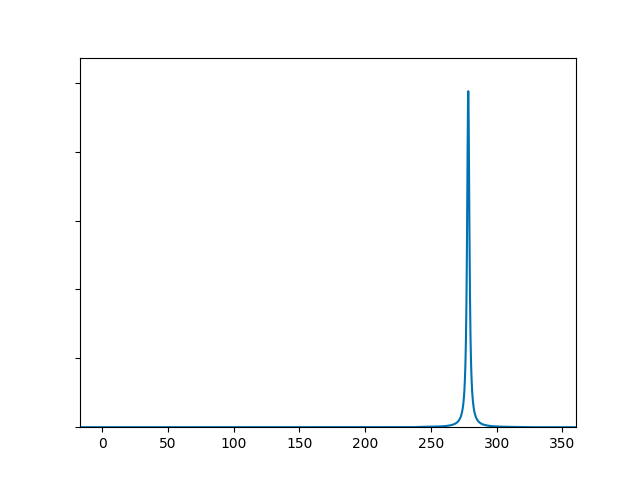Admittedly, it isn’t the most exciting spectrum, with a single peak around 280 cm-1. Let’s make some adjustments: we’ll add a touch more Gaussian broadening, zoom in on the peak by limiting the axis range, add axis labels and write to a file.

galore test/CaF2/ir_lda_700.txt -g 1.2 -l  --spikes \
--plot ir_lda_700_better.png \
--xmin 200 --xmax 350 --units cm-1 --ylabel Intensity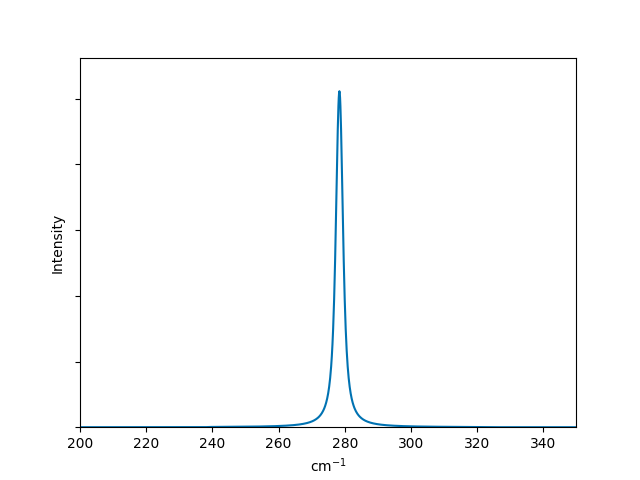Now the plot is more publication-ready! If you would like to use another plotting program, the broadened data can be output to a CSV file by simply replacing --plot with --csv:

galore test/CaF2/ir_lda_700.txt -g 1.2 -l -k --csv --xmin 200 --xmax 350


(Here we have also replaced --spikes with its short form -k.) This will write a csv file to the standard output as no filename was given. We can also write space-separated text data, so for example

galore test/CaF2/ir_lda_700.txt -g 1.2 -l -k --txt ir_CaF2_broadened.txt


generates a file with two columns (i.e. energy and broadened intensity).

## Simulated Raman¶

Broadening a simulated Raman spectrum is very similar to broadening a simulated IR spectrum. Galore recognises the output format of the vasp_raman.py code, which automates the process of following vibrational modes and calculating the polarisability change on each displacement. The output file has a simple format and Galore recognises them by inspecting the header. Sample data is included (computed with LDA using VASP with a 500 eV cutoff) as test/CaF2/raman_lda_500.dat. We generate a plot in the same way as before:

galore test/CaF2/raman_lda_500.dat -g -l -k --plot --units cm-1 --ylabel Intensity --style Solarized_Light2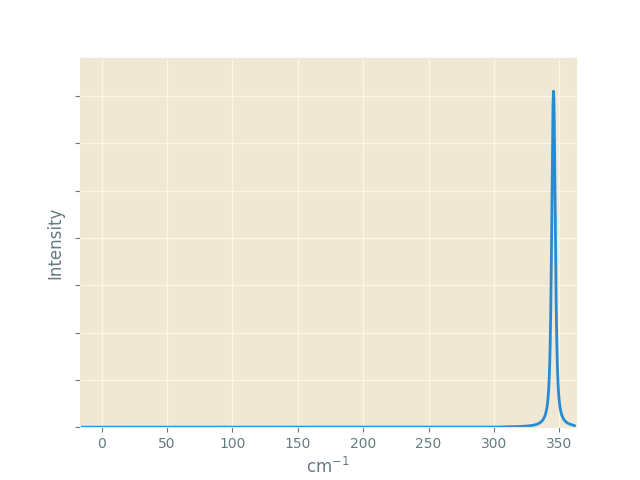This time we set an alternative appearance style with --style Solarized_Light2. Galore uses Matplotlib styles, and you can use inbuilt styles or create custom stylesheets. The dark_background style may be useful for slide presentations. Note that for the same material we are seeing a single peak again, but at a different frequency to the IR plot. This is not a shift; the peak at 280 cm-1 is still present but has zero activity, while the peak calculated at 345 cm-1 has zero IR activity.

## Simulated Photoelectron Spectroscopy¶

Photoelectron measurements allow valence band states to be probed fairly directly; energy is absorbed by an incident photon as it ejects an electron from the sample, and the shift in energy is measured relative to a monochromatic photon source. Ultraviolet photoelectron spectroscopy (UPS), x-ray photoelectron spectroscopy (XPS) and Hard x-ray photoelectron spectroscopy (HAXPES) are fundamentally similar techniques, differing in the energy range of the incident photons.

These binding energies may be compared with the full density of states (DOS) computed with ab initio methods. However, the intensity of interaction will vary depending on the character of the energy states and the energy of the radiation source. The relevant interaction parameter (“photoionization cross-section”) has been calculated systematically over the periodic table and relevant energy values; Galore includes some such data from Yeh and Lindau (1985).

In ab initio codes it is often possible to assign states to particular orbital characters; often this is limited to s-p-d-f (i.e. the second quantum number) but in principle an all-electron code can also assign the first quantum number. Directional character is also sometimes assigned, usually relative to Cartesian axes. These various schemes are used to construct a “projected density of states” (PDOS).

The construction of a PDOS in ab initio calculations is slightly arbitrary and lies beyond the scope of Galore. However, when the orbital assignment has been made the DOS elements can be weighted to simulate the photoionization spectrum.

We begin by plotting a PDOS from sample data in test/MgO. This was computed using VASP with standard pseudopotentials and the revTPSS exchange-correlation functional.

galore test/MgO/MgO_Mg_dos.dat test/MgO/MgO_O_dos.dat \
--plot --pdos -g 0.5 -l 0.2 --ylabel DOS --units eV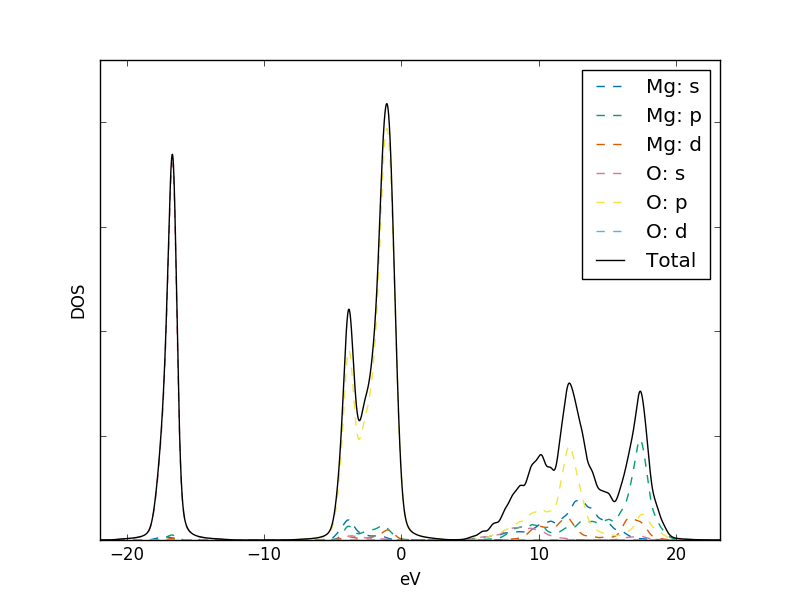Note that the --pdos flag is required to enable the reading of orbital-projected input files. The element identity is read from these filenames, and is expected between two underscore characters. The orbital names are determined from the column headers in this file. It is possible to use spin-polarised data by using a header form such as ‘s(up)’ and ‘s(down)’ for spin-polarised s-orbitals, in which case the contributions of the spin channels will be summed together.

Let’s turn this into a useful XPS plot. The flag --weightings can be used to pass a data file with cross-section data; for some cases data is build into Galore. Here we will use the data for Al k-α radiation which is denoted alka is built into Galore. We also flip the x-axis with --flipx to match the usual presentation of XPS data as positive ionisation or binding energies rather than the negative energy of the stable electron states. Finally the data is written to a CSV file with the --csv option.

galore test/MgO/MgO_Mg_dos.dat test/MgO/MgO_O_dos.dat \
--plot mgo_xps.png --pdos -g 0.2 -l 0.2 --weighting alka \
--units ev --xmin -1 --xmax 8 --ylabel Intensity \
--csv mgo_xps.csv --flipx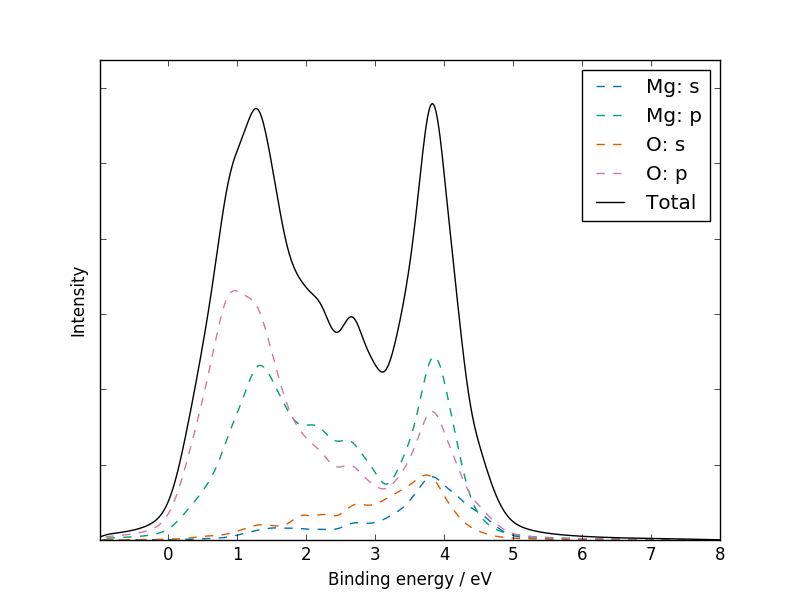Plotting the CSV file with a standard plotting package should give a similar result to the figure above; if not, please report this as a bug!

## Compare with literature: tin dioxide¶

Sample VASP output data is included for rutile tin dioxide. This was computed with the PBE0 functional, using a 4x4x5 k-point mesh and 700 eV basis-set cutoff. The structure was optimised to reduce forces to below 1E-3 eV Å-1 using 0.05 eV of Gaussian broadening and the DOS was computed on an automatic tetrahedron mesh with Blöchl corrections. Instead of the separate .dat files used above, we will take advantage of Galore’s ability to read a compressed vasprun.xml file directly. This requires the Pymatgen library to be installed:

pip3 install --user pymatgen


If the GPAW Python library is available, it is also possible to import this data from .gpw output files.

### XPS¶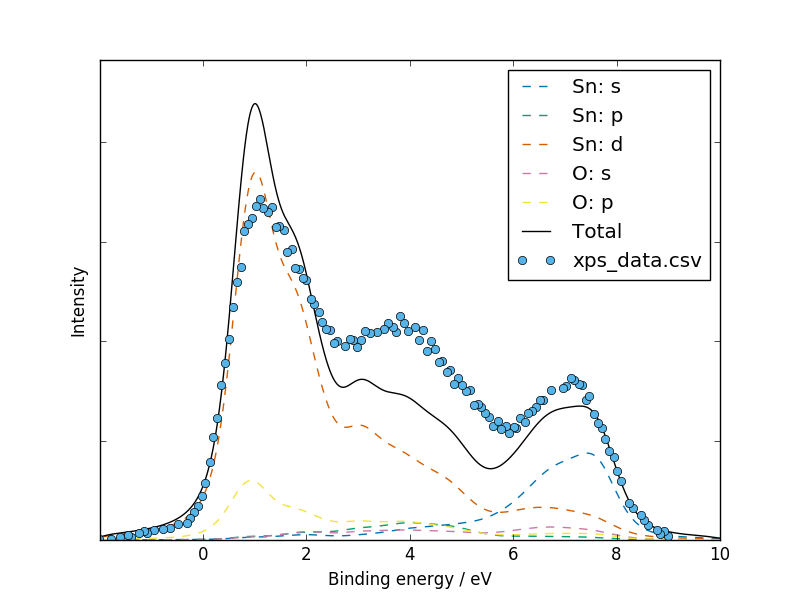We have digitised the experimental data plotted in Fig.3 of Farahani et al. (2014) in order to aid a direct comparison:

galore test/SnO2/vasprun.xml.gz --plot -g 0.5 -l 0.4 \
--pdos --w alka --flipx --xmin -2 --xmax 10 \
--overlay test/SnO2/xps_data.csv  --overlay_offset -4 \
--overlay_scale 5 --units ev --ylabel Intensity


(Note that here the shorter alias -w is used for the XPS weighting.) The general character and peak positions match well, but the relative peak intensities could be closer; the first peak is very strong even with cross-section weightings applied. We can see that the dip in between the second an third main peak corresponds to a crossing point between the contributions of the Sn-s and Sn-d orbitals.

A slightly more generous assignment of ‘p’ vs ‘d’ character by the orbital projection scheme would have made for a better fit! The published results seem to fit better despite using similar calculation parameters; we can’t see if the orbital breakdown is indeed the determining factor.

### UPS¶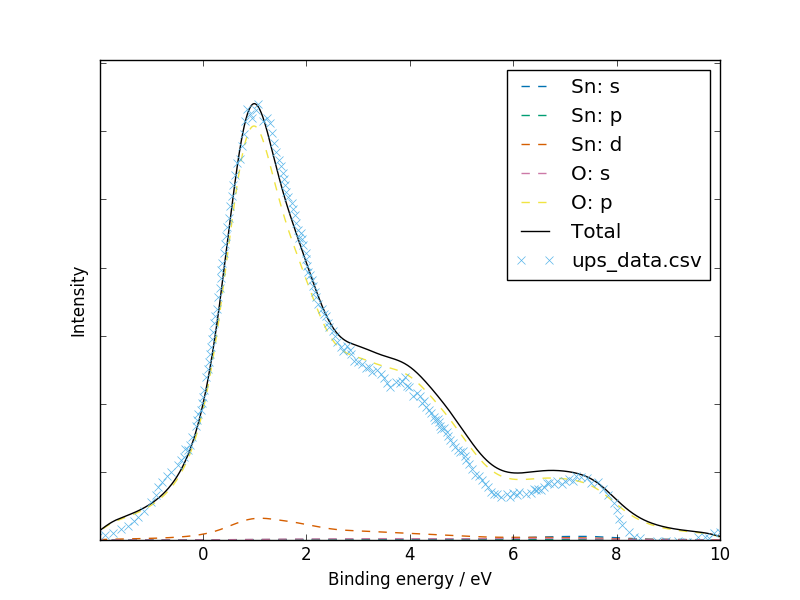Experimental UPS data was digitized from Fig. 1 of Themlin et al. (1990). A satisfactory fit is obtained for the three main peaks, but the “bump” below zero suggests the presence of some phenomenon in the bandgap which was not captured by the ab initio calculation:

galore test/SnO2/vasprun.xml.gz --plot -g 0.5 -l 0.9 \
--pdos --w he2 --flipx --xmin -2 --xmax 10 \
--overlay test/SnO2/ups_data.csv --overlay_offset 0.44 \
--units ev --ylabel Intensity --overlay_style x


The authors noted this in their own comparison to a DOS from tight-binding calculations:

The location of the VBM in out UPS data was complicated by the presence of a slowly varying photoelectron signal, resulting from a surface-state band.

### HAXPES¶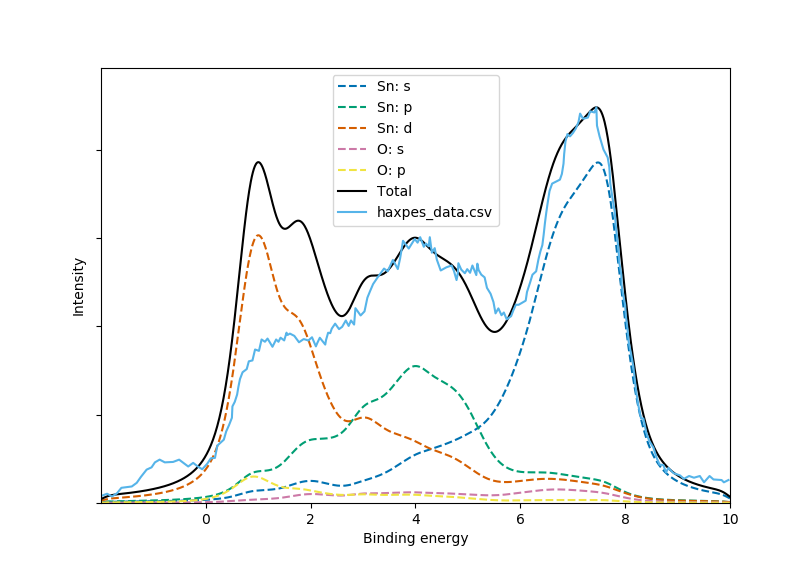A HAXPES spectrum was obtained by digitizing Fig. 1 of Nagata et al. (2011). These experiments were performed with 5.95 keV x-rays; we set --w 5.95 to estimate corresponding cross-sections by fitting to Scofield (1973):

galore test/SnO2/vasprun.xml.gz --plot -g 0.3 -l 0.5 --pdos \
--w 5.95 --flipx --xmin -2 --xmax 10 \
--overlay test/SnO2/haxpes_data.csv --overlay_offset -3.7 \
--ylabel Intensity --overlay_style -


We see that the weighting goes some way to rebalancing the peak intensities but once again the Sn-d states are over-represented. Surface states above the valence band are seen in the experimental data.

## Python API¶

The Python API allows custom plots to be produced by generating data sets Galore’s core functions and passing Matplotlib axes to the plotting functions. A few examples are provided below. It is not especially difficult to access the lines plotted to an axis and manipulate their appearance, rescale them etc.

### Overlaying different amounts of broadening¶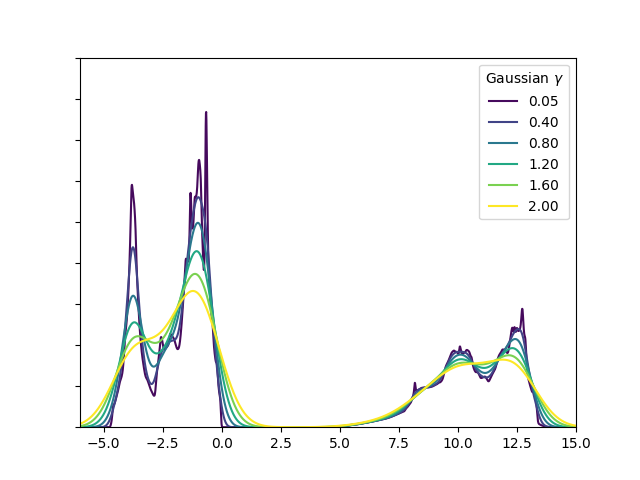1 2 3 4 5 6 7 8 9 10 11 12 13 14 15 16 17 18 19 20 21 22 23 24 25 26 27 28 29 30 31 32 33 34 #! /usr/bin/env python3 import numpy as np import matplotlib.pyplot as plt from matplotlib.cm import viridis as cmap import galore import galore.plot vasprun = 'test/MgO/vasprun.xml.gz' xmin, xmax = (-6, 15) fig = plt.figure() ax = fig.add_subplot(1, 1, 1) widths = np.arange(0, 2.01, 0.4) widths = 0.05 # Use a finite width in smallest case for g in widths: x_values, broadened_data = galore.process_1d_data(input=vasprun, gaussian=g, xmin=xmin, xmax=xmax) broadened_data /= g # Scale values by broadening width to conserve area galore.plot.plot_tdos(x_values, broadened_data, ax=ax) line = ax.lines[-1] line.set_label("{0:2.2f}".format(g)) line.set_color(cmap(g / max(widths))) ax.set_ylim(0, 1800) legend = ax.legend(loc='best') legend.set_title('Gaussian $\gamma$') plt.show() 

### Plotting different amounts of broadening on tiles¶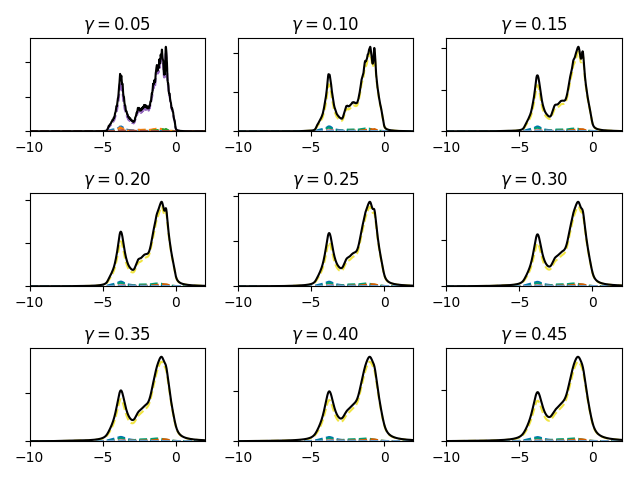1 2 3 4 5 6 7 8 9 10 11 12 13 14 15 16 17 18 19 20 21 22 23 #! /usr/bin/env python3 import numpy as np import matplotlib.pyplot as plt plt.style.use("seaborn-colorblind") import galore import galore.plot vasprun = './test/MgO/vasprun.xml.gz' xmin, xmax = (-10, 2) fig = plt.figure() for i, l in enumerate(np.arange(0.05, 0.50, 0.05)): ax = fig.add_subplot(3, 3, i + 1) ax.set_title("$\gamma = {0:4.2f}$".format(l)) plotting_data = galore.process_pdos(input=[vasprun], lorentzian=l, xmin=xmin, xmax=xmax) galore.plot.plot_pdos(plotting_data, ax=ax) ax.legend().set_visible(False) fig.tight_layout() plt.show() 

### Comparing different photoemission weightings¶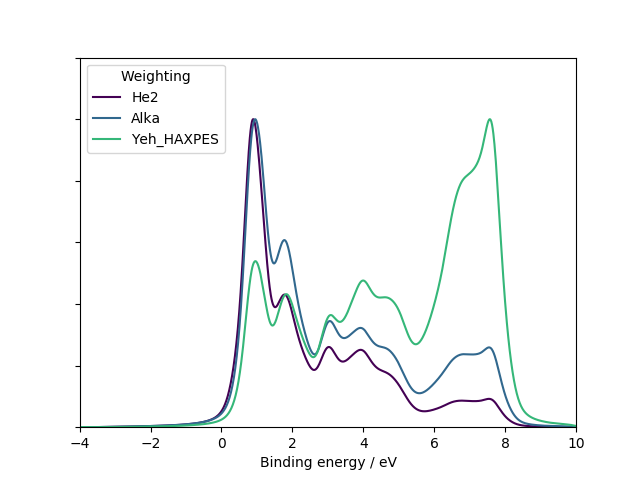1 2 3 4 5 6 7 8 9 10 11 12 13 14 15 16 17 18 19 20 21 22 23 24 25 26 27 28 29 30 31 32 33 34 35 36 #! /usr/bin/env python3 import numpy as np import matplotlib.pyplot as plt from matplotlib.cm import viridis as cmap import galore import galore.plot vasprun = 'test/SnO2/vasprun.xml.gz' xmin, xmax = (-10, 4) fig = plt.figure() ax = fig.add_subplot(1, 1, 1) weightings = ('He2', 'Alka', 'Yeh_HAXPES') for i, weighting in enumerate(weightings): plotting_data = galore.process_pdos(input=[vasprun], gaussian=0.3, lorentzian=0.2, xmin=xmin, xmax=xmax, weighting=weighting) galore.plot.plot_pdos(plotting_data, ax=ax, show_orbitals=False, units='eV', xmin=-xmin, xmax=-xmax, flipx=True) line = ax.lines[-1] line.set_label(weighting) line.set_color(cmap(i / len(weightings))) ymax = max(line.get_ydata()) line.set_data(line.get_xdata(), line.get_ydata() / ymax) ax.set_ylim((0, 1.2)) legend = ax.legend(loc='best') legend.set_title('Weighting') plt.show()